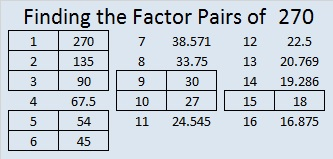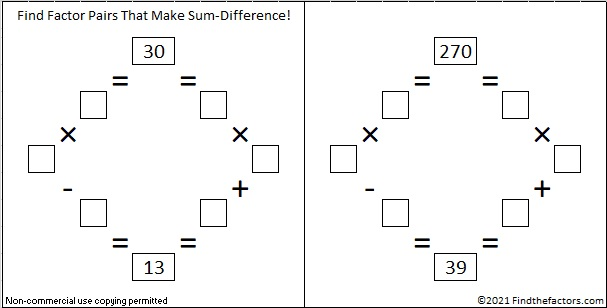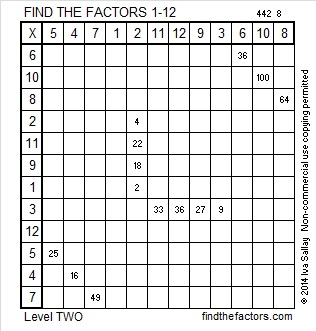# 270 and Level 2

Contents

### Today’s Puzzle:

Can you solve this level 2 puzzle?Print the puzzles or type the factors on this excel file: 12 Factors 2014-10-20

### Factors of 270:

• 270 is a composite number.
• Prime factorization: 270 = 2 x 3 x 3 x 3 x 5, which can be written 2 x (3^3) x 5
• The exponents in the prime factorization are 1, 3, and 1. Adding one to each and multiplying we get (1 + 1)(3 + 1)(1 + 1) = 2 x 4 x 2 = 16. Therefore 270 has 16 factors.
• Factors of 270: 1, 2, 3, 5, 6, 9, 10, 15, 18, 27, 30, 45, 54, 90, 135, 270
• Factor pairs: 270 = 1 x 270, 2 x 135, 3 x 90, 5 x 54, 6 x 45, 9 x 30, 10 x 27, or 15 x 18
• Taking the factor pair with the largest square number factor, we get √270 = (√9)(√30) = 3√30 ≈ 16.432### Sum-Difference Puzzles:

30 has four factor pairs. One of those factor pairs adds up to 13, and another subtracts to 13. Can you find those factor pairs to solve the first puzzle below?

270 has eight factor pairs. One of them adds up to 39, and a different one subtracts to 39. If you can identify those factor pairs, then you can solve the second puzzle!The second puzzle is really just the first puzzle in disguise. Why would I say that?

### Factors for Today’s Puzzle:This site uses Akismet to reduce spam. Learn how your comment data is processed.